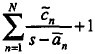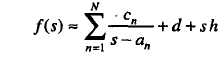40m QIL Cryo_Lab CTN SUS_Lab CAML OMC_Lab CRIME_Lab FEA ENG_Labs OptContFac Mariner WBEEShop
 SUS Lab eLog Not logged inMessage ID: 702     Entry time: Thu Aug 8 11:32:00 2013
 Author: Giorgos Type: Summary Category: SUS Subject: Compensation for Cross-Coupling and Vector Fitting

Cross-Coupling Measurements

Before measurig the cross-coupling effects, we put the plate back to our setup. Since the plate has magnets attached to it, we expect that it will affect the magnetic field produced by the coils. Therefore, a more realistic measurement of the cross-coupling effect that will resemble the real-time feedback control should take place in the presence of the plate. Of course, we want the plate to be still during the measurements, so that our Hall-effect sensors are not affected by their displacement (if the plate is still, it will only affect the reading of the sensors at 0 frequency, but we are looking at the transfer function over the whole frequency range). To fix the plate, we pressed all motors against it, ignoring any small fluctuations.

VECTOR FITTING

In this configuration, we used Diagnostic Tools software to measure the transfer function beween ACs coils' output and ACs sensors' reading. Then, we used Vector Fitting to obtain a fitting transfer function with zeros, poles, and gain that resembles the behavior of the cross-coupling. In few words, Vector Fitting uses a guess function σ(s)=, whose zeros are the poles of the desired function. Finding the zeros of σ(s) equals to finding the poles of the function and vector fitting manages to find a linear least-squares fit model by trying values for the poles and solving for the linear coefficients. More information can be found at http://www.sintef.no/Projectweb/VECTFIT/Algorithm/.

We developed a code for the vector fitting and found the best model with 20 pairs of poles (40 poles, since they are complex conjugates) and about 10 iterations. At the end, we applied compensation feedback given by the modelling transfer function so as to cancel the cross-coupling effects.Below, one can see the cross-coupling measurements before (left figure) and after (right figure) applying the modelled function. We modelled the coupling transfer functions only for ACi_Sensor - ACi_coil (i=1,2,3) pairs, because cross-coupling between e.g. AC1 coil and AC2 sensor was very small (as is evident in the figures). Using this technique, we minimized coupling effects from roughly -10dB to roughly -70dB.

ELOG V3.1.3-